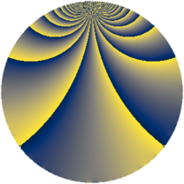# Properties

 Label 231.2.sLevel $231$ Weight $2$ Character orbit 231.s Rep. character $\chi_{231}(8,\cdot)$ Character field $\Q(\zeta_{10})$ Dimension $96$ Newform subspaces $1$ Sturm bound $64$ Trace bound $0$

# Related objects

## Defining parameters

 Level: $$N$$ $$=$$ $$231 = 3 \cdot 7 \cdot 11$$ Weight: $$k$$ $$=$$ $$2$$ Character orbit: $$[\chi]$$ $$=$$ 231.s (of order $$10$$ and degree $$4$$) Character conductor: $$\operatorname{cond}(\chi)$$ $$=$$ $$33$$ Character field: $$\Q(\zeta_{10})$$ Newform subspaces: $$1$$ Sturm bound: $$64$$ Trace bound: $$0$$

## Dimensions

The following table gives the dimensions of various subspaces of $$M_{2}(231, [\chi])$$.

Total New Old
Modular forms 144 96 48
Cusp forms 112 96 16
Eisenstein series 32 0 32

## Trace form

 $$96 q + 6 q^{3} - 24 q^{4} - 10 q^{6} - 20 q^{9} + O(q^{10})$$ $$96 q + 6 q^{3} - 24 q^{4} - 10 q^{6} - 20 q^{9} - 20 q^{12} - 32 q^{16} - 10 q^{18} - 60 q^{19} + 30 q^{24} + 12 q^{25} - 40 q^{28} + 60 q^{30} + 40 q^{31} - 44 q^{33} + 8 q^{34} + 2 q^{36} + 16 q^{37} - 10 q^{39} - 96 q^{45} - 40 q^{46} + 48 q^{48} + 24 q^{49} - 30 q^{51} - 40 q^{52} + 28 q^{55} - 30 q^{57} + 36 q^{58} - 32 q^{60} + 40 q^{61} - 28 q^{64} + 118 q^{66} - 56 q^{67} + 26 q^{69} + 20 q^{70} + 150 q^{72} + 40 q^{73} + 2 q^{75} - 20 q^{78} - 40 q^{79} + 8 q^{81} - 16 q^{82} + 40 q^{84} - 60 q^{85} - 156 q^{88} + 100 q^{90} + 36 q^{91} - 36 q^{93} + 160 q^{96} - 88 q^{97} - 94 q^{99} + O(q^{100})$$

## Decomposition of $$S_{2}^{\mathrm{new}}(231, [\chi])$$ into newform subspaces

Label Dim $A$ Field CM Traces $q$-expansion
$a_{2}$ $a_{3}$ $a_{5}$ $a_{7}$
231.2.s.a $96$ $1.845$ None $$0$$ $$6$$ $$0$$ $$0$$

## Decomposition of $$S_{2}^{\mathrm{old}}(231, [\chi])$$ into lower level spaces

$$S_{2}^{\mathrm{old}}(231, [\chi]) \cong$$ $$S_{2}^{\mathrm{new}}(33, [\chi])$$$$^{\oplus 2}$$